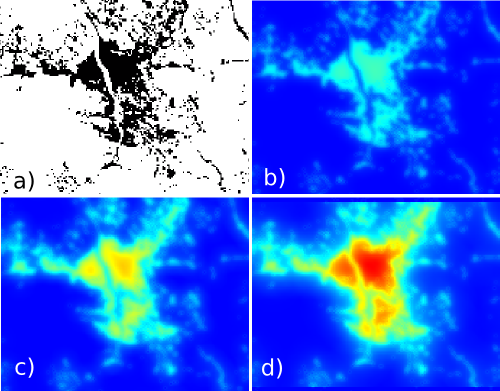## NAME

r.futures.devpressure - Module for computing development pressure

## KEYWORDS

raster, filter, statistics

## SYNOPSIS

r.futures.devpressure
r.futures.devpressure --help
r.futures.devpressure [-n] input=name output=name method=string size=integer [gamma=float] [scaling_factor=float] [--overwrite] [--help] [--verbose] [--quiet] [--ui]

### Flags:

-n
Do not propagate nulls
--overwrite
Allow output files to overwrite existing files
--help
Print usage summary
--verbose
Verbose module output
--quiet
Quiet module output
--ui
Force launching GUI dialog

### Parameters:

input=name [required]
Name of input binary raster map representing development
output=name [required]
Name of the output development pressure raster
method=string [required]
Method for computing development pressure
Options: occurrence, gravity, kernel
Default: gravity
occurrence: number of developed cells in window
gravity: scaling_factor / pow(distance, gamma)
kernel: scaling_factor * exp (-2*distance / gamma)
size=integer [required]
Half of neighborhood size
Default: 8
gamma=float
Coefficient controlling the influence of distance, needed for method gravity and kernel
Default: 1.5
scaling_factor=float
Scaling factor needed for method gravity and kernel
Default: 1

## DESCRIPTION

Module r.futures.devpressure is part of FUTURES, land change model. It computes development pressure, a predictor based on number of neighboring developed cells within search distance, weighted by distance. The development pressure variable plays a special role in the model, allowing for a feedback between predicted change and change in subsequent steps.

There are multiple options for calculating development pressure in the model.

• occurrence: simple count of the number of developed cells within the specified window size
• gravity: defined as scaling_factor / pow(distance, gamma)
• kernel: defined as scaling_factor * exp(-2 * distance / gamma)

Coefficient gamma is the coefficient controlling the influence of distance. The best value for gamma is chosen during the model selection process (TODO).

The input raster is binary, value 1 represents development, value 0 no development. The neighborhood size describes the size of the moving window in cells (2 * size + 1).Figure: Effect of parameters size and gamma: a) initial development, b) gamma = 2, size = 10, c) gamma = 1.5, size = 10, d) gamma = 1.5, size = 20.

## NOTES

By default NULL values are propagated. This means that the edges of resulting map will be NULLs. To avoid that, flag n internally enlarges the computational region, converts NULLs to zeros, performs the computation and then patches back the original NULL values.

Module r.futures.devpressure, although written for FUTURES model, is general enough to be used for different applications where distance pressure can be described with the functions above.

## EXAMPLES

```r.futures.devpressure input=developed output=pressure gamma=1.5 size=10 method=gravity
```

FUTURES, r.futures.pga, r.futures.calib, r.futures.demand, r.sample.category

## AUTHOR

Anna Petrasova, NCSU GeoForAll

Last changed: \$Date: 2018-09-14 21:49:22 -0400 (Fri, 14 Sep 2018) \$

## SOURCE CODE

Available at: r.futures.devpressure source code (history)

© 2003-2020 GRASS Development Team, GRASS GIS 7.8.4dev Reference Manual